Function Repository Resource:

# GlobalMaxima

Compute the global maxima of an expression with respect to the given variables

Contributed by: Wolfram|Alpha Math Team
 ResourceFunction["GlobalMaxima"][expr,x] computes the global maxima of expr with respect to x. ResourceFunction["GlobalMaxima"][expr,{x,y,…}] computes the global maxima of expr with respect to multiple variables. ResourceFunction["GlobalMaxima"][{expr,const},…] computes the global maxima of expr subject to the constraint const.

## Details and Options

ResourceFunction["GlobalMaxima"] returns a list with each maximum in the form {fmax,{xxmax,yymax,}}.
The const can contain equations, inequalities or logical combinations of these.
ResourceFunction["GlobalMaxima"] only returns results when there is a bounded maximum.

## Examples

### Basic Examples (2)

Compute the global maxima of a polynomial:

 In:=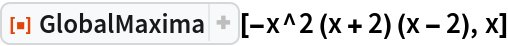Out=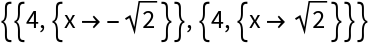Plot them:

 In:=Out=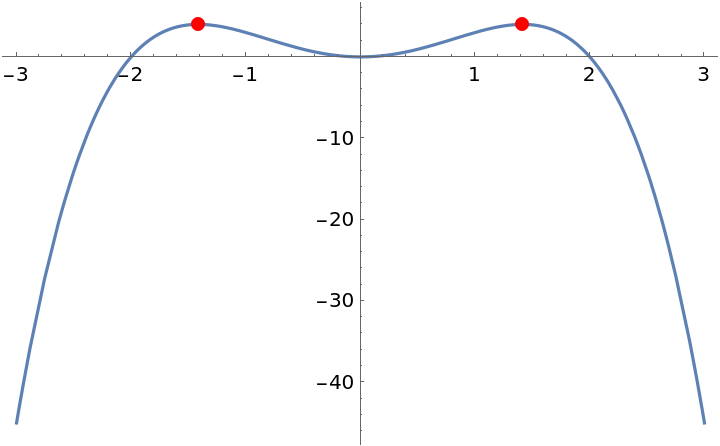### Scope (2)

Use a constraint in order to reduce the domain upon which maxima can be found:

 In:=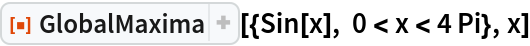Out=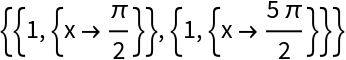Compute the maxima of a function of two variables:

 In:=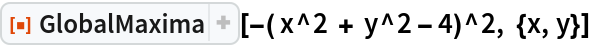Out=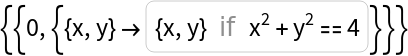### Possible Issues (1)

GlobalMaxima may return duplicate results for periodic functions:

 In:=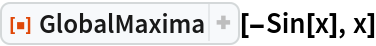Out=## Publisher

Wolfram|Alpha Math Team

## Version History

• 2.0.0 – 23 March 2023
• 1.0.0 – 18 September 2020

## Author Notes

To view the full source code for GlobalMaxima, run the following code:

 In:=